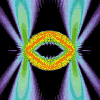• SearchHide Search
• Home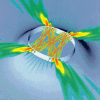• Vitae• News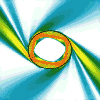• Papers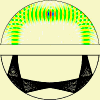• Microlasers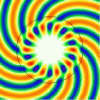• Research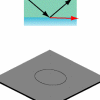• Teaching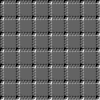• Address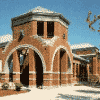# Dome resonators

## Mixed boundary conditions in dome cavities

### Exactly solvable problems

There are only a few optical resonator geometries for which the wave equation can be solved analytically in terms of special functions. An important and well-known example is the ideal dielectric sphere, for which the solution procedure (in the context of light scattering) was found by the German physicist Gustav Mie, then at the University of Greifswald (1908). The problem is solvable because the spherical surface coincides with an iso-surface of the spherical coordinate system, and the boundary conditions are rotationally invariant as well.

A new geometry for which at least the scalar wave equation can be solved analytically is the parabolic dome. This solution was obtained by me in collaboration with Isabelle Robert and Izo Abram (read more on a separate page: Ray and wave solutions in the parabolic dome). Here is a side view of a stationary state of the three-dimensional cavity, which has the shape of a dome: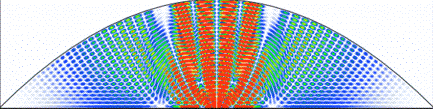This is an exact solution of the scalar wave equation. Some fully vectorial modes in such cavities can also be written down exactly. In contrast to the Mie problem, the boundaries here are not contour surfaces of any single orthogonal coordinate system. Moreover, the cavity has mixed boundary conditions if the top mirror is a perfect electric conductor while the bottom mirror is a dielectric mirror.

There is an extremely close connection between the exact wave solutions and the ray-optics approximation, which in this case turns out to yield quantitatively correct results for the cavity spectrum when combined with a standard semiclassical quantization approach.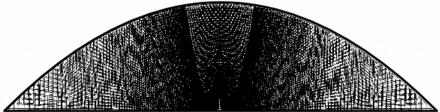Note the similarity between the above ray pattern and the wave plot above. The following link leads to additional material on this subject.

### Taking polarization seriously

Motivated by recent progress toward fabrication of dome cavities, my graduate student David Foster and I have taken this subject one step further: we study the fully vectorial modes of dome cavities with arbitrary shapes and various, mixed boundary conditons. This includes the effects of radiation losses through realistic Bragg mirrors, and is not limited to any paraxial approximation.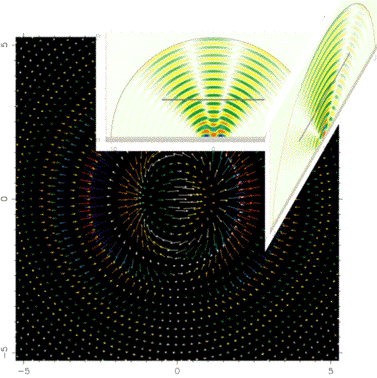Shown here is a cavity mode in two orthogonal side-view cross sections, together with its electric vector field plotted in a horizontal cross section whose location is indicated by the floating grey bars in the side views. Deviations from paraxiality then show up in the fact that the elctric field vector does not lie in a horizontal plane. The color in the vector plot indicates the magnitude of the vertical field components.

The methods used to perform these calculations, and some results, are reported in our paper: D. H. Foster and J. U. Nöckel, "Methods for 3-D vector microcavity problems involving a planar dielectric mirror", Optics Communications 234, 351-383 (2004).